## How to Calculate and Solve for Nernst Equation | Corrosion

The nernst equation is illustrated by the image below.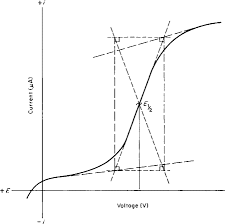To compute for nernst equation, four essential parameters are needed and these parameters are Electrochemical Cell Potential (ΔV°), Number of Electrons (n), Molar Concentration [M1n+] and Molar Concentration [M2n+].

The formula for calculating nernst equation:

ΔV = ΔV° – 0.0592/n log(M1n+/M2n+)

Where:

ΔV = Nernst Equation | Potential
ΔV° = Electrochemical Cell Potential
n = Number of Electrons
M1n+ = Molar Concentration
M2n+ = Molar Concentration

Given an example;
Find the nernst equation when the electrochemical cell potential is 3, the number of electrons is 9, the molar concentration is 6 and the molar concentration is 2.

This implies that;

ΔV° = Electrochemical Cell Potential = 3
n = Number of Electrons = 9
M1n+ = Molar Concentration = 6
M2n+ = Molar Concentration = 2

ΔV = ΔV° – 0.0592/n log(M1n+/M2n+)
ΔV = 3 – (0.0592/9) log(6/2)
ΔV = 3 – (0.00657) log (3)
ΔV = 3 – 0.0065 (0.477)
ΔV = 3 – (0.0031)
ΔV = 2.99

Therefore, the nernst equation is 2.99 V.

## How to Calculate and Solve for Nernst Equation | Corrosion

The nernst equation is illustrated by the image below.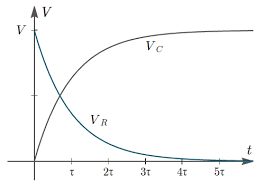To compute for nernst equation, seven essential parameters are needed and these parameters are Electrochemical Cell Potential (ΔV°), Gas Constant (R), Temperature (T), Number of Electrons (n), Faraday’s Constant (F), Molar Concentration [M1n+] and Molar Concentration [M2n+].

The formula for caluclating nernst equation:

ΔV = ΔV° – RT/nF In(M1n+/M2n+)

Where:

ΔV = Nernst Equation | Potential
ΔV° = Electrochemical Cell Potential
R = Gas Constant
T = Temperature
n = Number of Electrons
M1n+ = Molar Concentration
M2n+ = Molar Concentration

Let’s solve an example;
Find the nernst equation when the electrochemical cell potential is 8, the gas constant is 2, the temperature is 5, the number of electrons is 3, the faraday’s constant is 9, the molar concentration is 10 and the molar concentration is 4.

This implies that;

ΔV° = Electrochemical Cell Potential = 8
R = Gas Constant = 2
T = Temperature = 5
n = Number of Electrons = 3
F = Faraday’s Constant = 9
M1n+ = Molar Concentration = 10
M2n+ = Molar Concentration = 4

ΔV = ΔV° – RT/nF In(M1n+/M2n+)
ΔV = 8 – ((2)(5)/(3)(9)) In(10/4)
ΔV = 8 – (10/27) In (2.5)
ΔV = 8 – 0.37 (0.91)
ΔV = 8 – (0.339)
ΔV = 7.66

Therefore, the nernest equation is 7.66 V.

## How to Calculate and Solve for Electrochemical Cell Potential | Corrosion

The electrochemical cell potential is illustrated by the image below.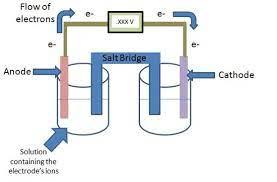To compute for electrochemical cell potential, two essential parameters are needed and these parameters are Positive Cell Potential (V2°) and Negative Cell Potential (V1°).

The formula for calculating electrochemical cell potential:

ΔV° = V2° – V1°

Where:

ΔV° = Electrochemical Cell Potential
V2° = Positive Cell Potential
V1° = Negative Cell Potential

Given an example;
Find the electrochemical cell potential when the positive cell potential is 14 and the negative cell potential is 7.

This implies that;

V2° = Positive Cell Potential = 14
V1° = Negative Cell Potential = 7

ΔV° = V2° – V1°
ΔV° = 14 – 7
ΔV° = 7

Therefore, the elecreochemical cell potential is 7 V.

Calculating the Positive Cell Potential when the Electrochemical Cell Potential and the Negative Cell Potential is Given.

V2° = ΔV° + V1°

Where:

V2° = Positive Cell Potential
ΔV° = Electrochemical Cell Potential
V1° = Negative Cell Potential

Let’s solve an example;
Find the positive cell potential when the electrochemical cell potential is 20 and the negative cell potential is 14.

This implies that;

ΔV° = Electrochemical Cell Potential = 20
V1° = Negative Cell Potential = 14

V2° = ΔV° + V1°
V2° = 20 + 14
V2° = 34

Therefore, the positive cell potential is 34 V.

## How to Calculate and Solve for Moisture Content | Refractories

The moisture content is illustrated by the image below.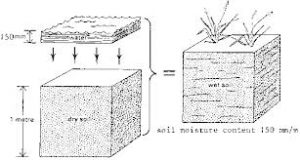To compute for moisture content, two essential parameters are needed and these parameters are Weight of the Sample before Drying (G) and Weight of the Sample after Drying (G1).

The formula for calculating moisture content:

w = 100((G – G1)/G)

Where:

w = Moisture Content
G = Weight of the Sample before Drying
G1 = Weight of the Sample after Drying

Let’s solve an example;
Find the moisture content when the weight of the sample before drying is 40 and the weight of the sample after drying is 10.

This implies that;

G = Weight of the Sample before Drying = 40
G1 = Weight of the Sample after Drying = 10

w = 100((G – G1)/G)
w = 100((40 – 10)/40)
w = 100(30/40)
w = 100(0.75)
w = 75

Therefore, the moisture content is 75%.

## How to Calculate and Solve for Loss on Ignition | Refractories

The loss on ignition is illustrated by the image below.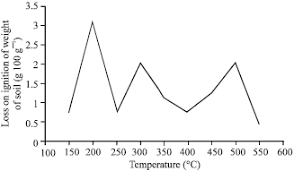To compute for loss on ignition, two essential parameters are needed and these parameters are Weight before Firing (m1) and Weight after Firing (m2).

The formula for calculating loss on ignition:

LOI = 100((m1 – m2)/m1)

Where:

LOI = Loss On Ignition
m1 = Weight before Firing
m2 = Weight after Firing

Let’s solve an example;
Find the loss on ignition when the weight before firing is 22 and the weight after firing is 11.

This implies that;

m1 = Weight before Firing = 22
m2 = Weight after Firing = 11

LOI = 100((m1 – m2)/m1)
LOI = 100((22 – 11)/22)
LOI = 100(11/22)
LOI = 100(0.5)
LOI = 50

Therefore, the loss on ignition is 50%.

## How to Calculate and Solve for Apparent Porosity | Refractories

The apparent porosity is illustrated by the image below.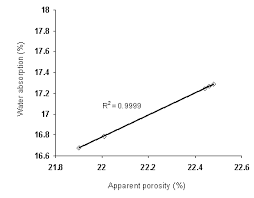To compute for apparent porosity, three essential parameters are needed and these parameters are Soaked Weight (W), Dried Weight (D) and Suspended Weight (S).

The formula for calulating apparent porosity:

AP = 100((W – D)/(W – S))

Where:

AP = Apparent Porosity
W = Soaked Weight
D = Dried Weight
S = Suspended Weight

Let’s solve an example;
Find the apparent porosity when the soaked weight is 14, the dried weight is 10 and the suspended weight is 12.

This implies that;

W = Soaked Weight = 14
D = Dried Weight = 10
S = Suspended Weight = 12

AP = 100((W – D)/(W – S))
AP = 100((14 – 10)/(14 – 12))
AP = 100(4/2)
AP = 100(2)
AP = 200

Therefore, the apparent porosity is 200%.

## How to Calculate and Solve for Bulk Density | Refractories

The bulk density is illustrated by the image below.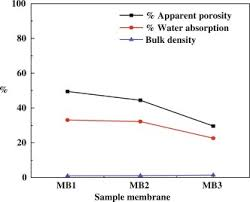To compute for bulk density, four essential parameters are needed and these parameters are Dried Weight (W), Density of Water (ρw), Soaked Weight (W) and Suspended Weight (S).

The formula for calculating bulk density:

BD = w/W – S

Where:

BD = Bulk Density
D = Dried Weight
ρw = Density of Water
W = Soaked Weight
S = Suspended Weight

Let’s solve an example;
Find the bulk density when the dried weight is 6, the density of water is 10, the soaked weight is 14 and the suspended weight is 9.

This implies that;

D = Dried Weight = 6
ρw = Density of Water = 10
W = Soaked Weight = 14
S = Suspended Weight = 9

BD = w/W – S
BD = (6)(10)/(14 – 9)
BD = (60)/(5)
BD = 12

Therefore, the bulk density is 12g/cm³.

Calculating the Dried Weight when the Bulk Density, the Density of Water, the Soaked Weight and the Suspended Weight is Given.

D = BD(W – S) / ρw

Where:

D = Dried Weight
BD = Bulk Density
ρw = Density of Water
W = Soaked Weight
S = Suspended Weight

Let’s solve an example;
Find the dried weight when the bulk density is 10, the density of water is 4, the soaked weight is 8 and the suspended weight is 5.

This implies that;

BD = Bulk Density = 10
ρw = Density of Water = 4
W = Soaked Weight = 8
S = Suspended Weight = 5

D = BD(W – S) / ρw
D = 10(8 – 5) / 4
D = 10(3) / 4
D = 30 / 4
D = 7.5

Therefore, the dried weight is 7.5g.

## How to Calculate and Solve for Fired Linear Shrinkage | Refractories

The fired linear shrinkage is illustrated by the image below.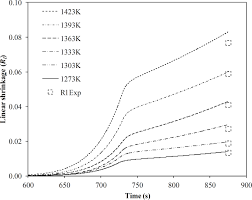To compute for fired linear shrinkage, two essential parameters are needed and these parameters are Dried Length (DL) and Fired Length (FL).

The formula for calculating fired linear shrinkage:

FLS = 100(DL – FL/DL)

Where:

FLS = Fired Linear Shrinkage
DL = Dried Length
FL = Fired Length

Given an example;
Find the fired linear shrinkage when the dried length is 32 and the fired length is 12.

This implies that;

DL = Dried Length = 32
FL = Fired Length = 12

FLS = 100(DL – FL/DL)
FLS = 100((32 – 12)/32)
FLS = 100(20/32)
FLS = 100(0.625)
FLS = 62.5

Therefore, the fired linear shrinkage is 62.5%.

## How to Calculate and Solve for Cold Crushing Strength | Refractories

The cold crushing strength is illustrated by the image below.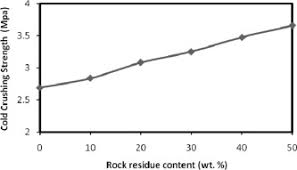To compute for cold crushing strength, two essential parameters are needed and these parameters are Maximum Load (MI) and Cross-sectional Area (A).

The formula for calculating cold crushing strength:

CCS = MI/A

Where:

CCS = Cold Crushing Strength
A = Cross-sectional Area

Let’s solve an example;
Find the cold crushing strength when the maximum load is 44 and the cross-sectional area is 11.

This implies that;

MI = Maximum Load = 44
A = Cross-sectional Area = 11

CCS = MI/A
CCS = 44/11
CCS = 4

Therefore, the cold crushing strength is 4 Pa.

Calculating the Maximum Load when the Cold Crushing Strength and the Cross-Sectional Area is Given.

MI = CCS x A

Where:

CCS = Cold Crushing Strength
A = Cross-sectional Area

Let’s solve an example;
Given that the cold crushing strength is 15 and the cross-sectional area is 5. Find the maximum load?

This implies that;

CCS = Cold Crushing Strength = 15
A = Cross-sectional Area = 5

MI = CCS x A
MI = 15 x 5
MI = 75

Therefore, the cold crushing strength is 75.

## How to Calculate and Solve for Permeability Number | Refractories

The permeability number is illustrated by the image below.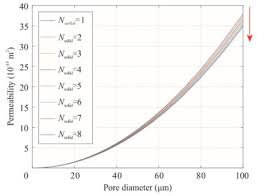To compute for the permeability number, five essential parameters are needed and these parameters are Volume of Air (V), Height of the Specimen (H), Cross-sectional Area (A), Air Pressure (P) and Time (t).

The formula for calculating permeability number:

PN = VH/APt

Where:

PN = Permeability Number
V = Volume of Air
H = Height of the Specimen
A = Cross-sectional Area
P = Air Pressure
t = Time

Let’s solve an example;
Find the permeability number when the volume of air is 2, the height of the specimen is 7, the cross-sectional area is 3, the air pressure is 9 and the time is 4.

This implies that;

V = Volume of Air = 2
H = Height of the Specimen = 7
A = Cross-sectional Area = 3
P = Air Pressure = 9
t = Time = 4

PN = VH/APt
PN = (2)(7)/(3)(9)(4)
PN = (14)/(108)
PN = 0.129

Therefore, the permeability number is 0.129.